https://doi.org/10.5194/tc-14-811-2020
https://doi.org/10.5194/tc-14-811-2020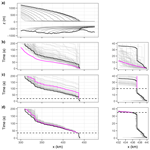# Assimilation of surface observations in a transient marine ice sheet model using an ensemble Kalman filter

Fabien Gillet-Chaulet
Abstract

Marine-based sectors of the Antarctic Ice Sheet are increasingly contributing to sea level rise. The basal conditions exert an important control on the ice dynamics and can be propitious to instabilities in the grounding line position. Because the force balance is non-inertial, most ice flow models are now equipped with time-independent inverse methods to constrain the basal conditions from observed surface velocities. However, transient simulations starting from this initial state usually suffer from inconsistencies and are not able to reproduce observed trends. Here, using a synthetic flow line experiment, we assess the performance of an ensemble Kalman filter for the assimilation of transient observations of surface elevation and velocities in a marine ice sheet model. The model solves the shallow shelf equation for the force balance and the continuity equation for ice thickness evolution. The position of the grounding line is determined by the floatation criterion. The filter analysis estimates both the state of the model, represented by the surface elevation, and the basal conditions, with the simultaneous inversion of the basal friction and topography. The idealised experiment reproduces a marine ice sheet that is in the early stage of an unstable retreat. Using observation frequencies and uncertainties consistent with current observing systems, we find that the filter allows the accurate recovery of both the basal friction and topography after few assimilation cycles with relatively small ensemble sizes. In addition it is found that assimilating the surface observations has a positive impact on constraining the evolution of the grounding line during the assimilation window. Using the initialised state to perform century-scale forecast simulations, we show that grounding line retreat rates are in agreement with the reference; however remaining uncertainties in the basal conditions may lead to significant delays in the initiation of the unstable retreat. These results are encouraging for the application to real glacial systems.

Share
Dates
1 Introduction

Despite recent significant improvements in ice sheet models, the projected magnitude and rate of the Antarctic and Greenland ice sheets' contribution to 21st century sea-level rise (SLR) remains poorly constrained . Improving our ability to model the century-scale magnitude and rates of mass loss from marine ice sheets remains a key scientific objective .

Improving SLR estimates requires, amongst other things, correctly modelling the dynamics of the grounding line (GL), i.e. the location where the ice detaches from its underlying bed and goes afloat on the ocean . In the GL vicinity, the stress regime changes from a regime dominated by vertical shearing in the grounded part to a buoyancy-driven flow dominated by longitudinal stretching and lateral shearing . Because this transition occurs on horizontal dimensions that are smaller than the typical grid size of large-scale ice sheet models, many studies have focussed on the ability of the numerical model to properly simulate grounding line migration using synthetic experiments . Two Marine Ice Sheet Model Intercomparison Projects (MISMIP) have allowed the identification of the minimum requirements to properly resolve GL motion: (i) inclusion of membrane stresses and (ii) a sufficiently small grid size or a subgrid interpolation of the GL . These results suggest that, in realistic applications, the numerical error could be reduced below the errors associated with uncertainties in the initial model state, in the model parameters, and in the forcings from the atmosphere and ocean.

For obvious reasons of inaccessibility, the basal conditions (topography and friction) are an important source of uncertainties. Because of the intrinsic instability of marine ice sheets resting over a seaward up‐sloping bed, the resolution of the bed topography in the coastal regions can significantly affect short-term ice sheet forecasts . Analytical developments have shown that the flux at the grounding line depends on the friction law and its coefficients . The sensitivity of model projections to the basal friction has been confirmed by several numerical studies on both synthetic and real applications . In particular, have shown that, for unbuttressed ice sheets, spatially varying friction coefficients can also lead to stable GL positions in up‐sloping bed regions.

Uncertainties in the model state and parameters can be reduced by data assimilation (DA). The objective of formal DA methods is to update the model using observations in a framework consistent with the model, the data and their associated uncertainties . Most ice flow models are now equipped with variational methods to constrain the basal conditions from surface observations . However most studies perform “snapshot” calibrations, where the inversion is performed at a unique initial time step. The state of the model produced from this calibration is therefore sensitive to inconsistencies between the different datasets. The resulting transient artefacts are usually dissipated during a relaxation period where the model drifts from the observations.

Because historic remote sensing data collections are spatially incomplete as well as temporally sparse, most distributed maps are mosaicked, stacked or averaged to maximise the spatial coverage at the expense of the temporal information . However, in the last few years, the development of space-borne ice sheet observations has entered a new era with the launch of new satellite missions, considerably increasing the spatial and temporal resolution of surface observations. Because they require linearised versions of the forecast model and of the observation operator, extending the existing variational methods implies important numerical developments . In , a time-dependent adjoint ice flow model is derived using a source-to-source algorithmic differentiation software combined with analytical methods. The DA capabilities are illustrated with a suite of synthetic experiments, including the simultaneous inversion of the basal topography and friction from surface observations and the assimilation of transient surface elevations to retrieve initial ice thicknesses. In a real-world application to a region of West Antarctica, they show that assimilating annually resolved observations of surface height and velocities between 2002 and 2011 allows the improvement of the initial model state, giving better confidences in projected committed mass losses . Because of the complexity of the code, use an operator-overloading approach to generate the adjoint and assimilate surface altimetry observations from 2003 to 2009 to constrain the temporal evolution of the basal friction and surface mass balance of the Northeast Greenland Ice Stream.

Ensemble DA methods, based on the ensemble Kalman filter (EnKF), have been successful in solving DA problems with large and non-linear geophysical models. Comparative discussions of the performances and advantages of variational and ensemble DA methods can be found in, e.g. , and . As they aim at solving similar problems, a recent tendency is to combine both methods to benefit from their respective advantages.

EnKF approximates the state and the error covariance matrix of a system using an ensemble that is propagated forward in time with the model, avoiding the computation of the covariance matrices and the use of linearised or adjoint models. Contrary to time-dependent variational methods where the objective is to find the model trajectory that minimises the difference with all the observations within an assimilation window, EnKF assimilates the observations sequentially in time as they become available using the analysis step of the Kalman filter, as illustrated in Fig. 1. The model trajectory is then discontinuous and, at a given analysis, the model is only informed by past and present observations. For the retrospective analysis of a time period in the past, i.e. a reanalysis, ensemble filters can easily be extended to smoothers to provide analyses that are informed by all past, present and future observations . Since the first version introduced by , many variants have been developed, mainly differing in the way the Kalman filter analysis is rewritten and the analysed error covariance matrix is resampled . A review of the most popular EnKFs using common notations can be found in . Efficient and parallel algorithms have been developed, and because they are independent of the forward model, several open-source toolboxes that implement various EnKFs are now available, e.g. OpenDA (https://www.openda.org, last access: 25 June 2018) and PDAF (http://pdaf.awi.de, last access: 25 June 2018).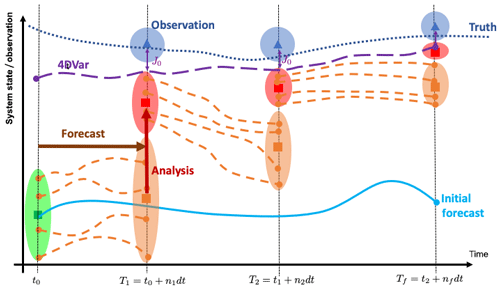Figure 1Principle of data assimilation (adapted from ). Having a physical model able to forecast the evolution of a system from time t=t0 to time t=Tf (cyan curve), the aim of DA is to use available observations (blue triangles) to correct the model projections and get closer to the (unknown) truth (dotted line). In EnKFs, the initial system state and its uncertainty (green square and ellipsoid) are represented by Ne members. The members are propagated forward in time during n1 model time steps dt to t=T1 where observations are available (forecast phase, orange dashed lines). At T=t1 the analysis uses the observations and their uncertainty (blue triangle and ellipsoid) to produce a new system state that is closer to the observations and with a lower uncertainty (red square and ellipsoid). A new forecast is issued from the analysed state and this procedure is repeated until the end of the assimilation window at t=Tf. The model state should get closer to the truth and with lower uncertainty as more observations are assimilated. Time-dependent variational methods (4D-Var) iterate over the assimilation window to find the trajectory that minimises the misfit (J0) between the model and all observations available from t0 to Tf (violet curve). For linear dynamics, Gaussian errors and infinite ensemble sizes, the states produced at the end of the assimilation window by the two methods should be equivalent .

As Monte Carlo methods, EnKFs suffer from under-sampling issues as often the size of the ensemble is much smaller than the size of the system to estimate. Localisation and inflation are popular methods to counteract these issues and to increase the stability of the filtering. Because they are based on the original Kalman filter equations, EnKFs are optimal only for Gaussian distributions and linear models. However, the many applications in geoscience with large and non-linear models have shown that the method remains robust in general and EnKFs are used in several operational centres with atmosphere, ocean and hydrology models . While firstly developed for numerical weather and ocean prediction where the forecasts are very sensitive to the model initial state, the method is also widely used, e.g. in hydrology, for joint state and parameter estimations .

In the context of ice sheet modelling, encouraging results have been obtained by for the estimation of the state and basal conditions of an ice sheet model using the ensemble transform Kalman filter (ETKF; Bishop et al.2001; Hunt et al.2007). They study the performance of the method using idealised twin experiments where perturbed observations generated from a model run are used in the DA framework to retrieve the true model states and parameters. Using a flow line shallow ice model, they show that both the basal topography and basal friction can be retrieved with good accuracy from surface observations with realistic noise levels, even for relatively small ensembles. The method has been further developed to assimilate the margin position in a shallow ice model that explicitly tracks the boundaries with a moving mesh method .

The purpose of this paper is to explore the performance of ensemble Kalman filtering for the initialisation of a marine ice sheet model that includes GL migration. In particular, we want to address (i) the quality of the analysis for the simultaneous estimation of the basal topography and friction in the context of a marine ice sheet that is undergoing an unstable GL retreat and (ii) the effects of the remaining uncertainties for the predictability of GL retreat. The ice flow model and the EnKF used in this study are described in Sect. 2. To test the DA framework, we define a twin experiment in Sect. 3. Section 4 presents the results for both the transient assimilation and the forecasts. Finally, perspectives and challenges for real applications are discussed in Sect. 5, before concluding remarks.

2 Methods

## 2.1 Ice flow model

The gravity-driven free surface flow of ice is solved using the finite-element ice flow model Elmer/Ice .

For the force balance, we solve the shelfy stream approximation (SSA) equation (MacAyeal1989) in one horizontal dimension. This is a vertically integrated model that derives from the Stokes equations for small aspect ratio and basal friction. In 1D, this leads to the following non-linear partial differential equation for the horizontal velocity field u:

$\begin{array}{}\text{(1)}& \frac{\partial }{\partial x}\left(\mathrm{4}\stackrel{\mathrm{‾}}{\mathit{\eta }}H\frac{\partial u}{\partial x}\right)-{\mathit{\tau }}_{\mathrm{b}}={\mathit{\rho }}_{\mathrm{i}}gH\frac{\partial {z}_{\mathrm{s}}}{\partial x},\end{array}$

with ρi the ice density, g the gravity norm, H=zszb the ice thickness, and zs and zb the top and bottom surface elevations, respectively. Using Glen's constitutive flow law, the vertically averaged effective viscosity $\stackrel{\mathrm{‾}}{\mathit{\eta }}$ is given by

$\begin{array}{}\text{(2)}& \stackrel{\mathrm{‾}}{\mathit{\eta }}=\frac{\mathrm{1}}{H}\underset{{z}_{\mathrm{b}}}{\overset{{z}_{\mathrm{s}}}{\int }}\frac{\mathrm{1}}{\mathrm{2}}{A}^{-\mathrm{1}/n}{D}_{\mathrm{e}}^{\left(\mathrm{1}-n\right)/n}\text{d}z,\end{array}$

where De is the second invariant of the strain-rate tensor, here equal to ${D}_{\mathrm{e}}^{\mathrm{2}}=\left(\partial u/\partial x{\right)}^{\mathrm{2}}$, A is the rate factor and n is the creep exponent, taken equal to the usual value n=3 in the following. The basal friction τb is null under floating ice and is represented by the non-linear Weertman friction law for grounded ice:

$\begin{array}{}\text{(3)}& {\mathit{\tau }}_{\mathrm{b}}=C{u}^{m},\end{array}$

with C and m the friction coefficient and exponent, respectively. In the following, we use the classical power law with $m=\mathrm{1}/n=\mathrm{1}/\mathrm{3}$. When in contact with the ocean, the ice is assumed to be in hydrostatic equilibrium. The floating condition is evaluated directly at the integration points, and τb in Eq. (1) is set to 0 wherever ice is floating .

The time dependency is introduced by the evolution of the top and bottom free surfaces. Because of the hydrostatic equilibrium, the ice sheet topography is fully defined by the bed elevation b and only one prognostic variable. Equation (1) is then coupled with the vertically integrated mass conservation equation for the evolution of the ice thickness H:

$\begin{array}{}\text{(4)}& \frac{\partial H}{\partial t}+\frac{\partial \left(uH\right)}{\partial x}={a}_{\mathrm{s}}-{a}_{\mathrm{b}},\end{array}$

with as the surface accumulation rate and ab the basal melt rate. The free surfaces zs and zb are obtained from the floating condition which, for zs, using a constant sea level zsl=0, gives

with ρw the sea water density.

## 2.2 Data assimilation

### 2.2.1 Filter algorithm

For the assimilation, we use the error subspace ensemble transform Kalman filter (ESTKF; Nerger et al.2012). Originally derived from the singular evolutive interpolated Kalman filter (SEIK; Pham et al.1998), ESTKF leads to the same ensemble transformations as the ETKF but at a slightly lower computational cost. In practice we use the local version of the filter implemented in PDAF (http://pdaf.awi.de, last access: 25 June 2018; ) and coupled to Elmer/Ice in an offline mode. This section outlines the ESTKF algorithm.

As an EnKF, ESTKF approximates the state xk and the error covariance matrix Pk of a system at time tk using an ensemble of Ne realisations ${\mathbit{x}}_{i}^{k}$, $i=\mathrm{1},\mathrm{\dots },{N}_{\mathrm{e}}$. The state vector, of size Nx, contains the prognostic variables and model parameters to be estimated and is approximated by the ensemble mean,

$\begin{array}{}\text{(6)}& {\stackrel{\mathrm{‾}}{\mathbit{x}}}^{k}=\frac{\mathrm{1}}{{N}_{\mathrm{e}}}\sum _{i=\mathrm{1}}^{{N}_{\mathrm{e}}}{\mathbit{x}}_{i}^{k},\end{array}$

while the error covariance matrix is approximated by

$\begin{array}{}\text{(7)}& {\mathbf{P}}_{k}=\frac{\mathrm{1}}{{N}_{\mathrm{e}}-\mathrm{1}}{{\mathbf{X}}^{\prime }}_{k}{{\mathbf{X}}^{\prime }}_{k}^{T},\end{array}$

where ${{\mathbf{X}}^{\prime }}_{k}=\left({\mathbit{x}}_{\mathrm{1}}^{k}-{\stackrel{\mathrm{‾}}{\mathbit{x}}}^{k},\mathrm{\dots },{\mathbit{x}}_{{N}_{\mathrm{e}}}^{k}-{\stackrel{\mathrm{‾}}{\mathbit{x}}}^{k}\right)\in {\mathbb{R}}^{{N}_{x}×{N}_{\mathrm{e}}}$ is the ensemble perturbation matrix.

The algorithm can be decomposed in two steps, the forecast and the analysis. Superscripts f (a) denote quantities related to each step. The forecast propagates the state and the error covariance matrix of the system forward in time, from a previous analysis at $t={t}_{k-\mathrm{1}}$ to the next observation time t=tk. For this, the numerical model k, assumed perfect in the sequel, is used to propagate each ensemble member individually during ndt model time steps:

$\begin{array}{}\text{(8)}& {\mathbit{x}}_{i}^{f,k}={\mathcal{M}}_{k}\left({\mathbit{x}}_{i}^{a,k-\mathrm{1}}\right).\end{array}$

At t=tk, a vector of observations ${\mathbit{y}}_{\mathrm{o}}^{k}$ of size Ny (usually with NyNx) is available. ${\mathbit{y}}_{\mathrm{o}}^{k}$ is related to the true system state xf by ${\mathbit{y}}_{\mathrm{o}}^{k}=\mathcal{H}\left({\mathbit{x}}^{f}\right)+{\mathbit{ϵ}}^{k}$, where the observation error ϵk is assumed to be a white Gaussian distributed process with known covariance matrix Rk, and is the observation operator that relates the state variables to the observations. When ${\mathbit{y}}_{\mathrm{o}}^{k}$ is the observed surface velocities, the relation between the observations and the system state, i.e., the ice sheet geometry, and parameters, i.e. the boundary conditions, is given by the force balance Eq. (1); thus is a non-linear elliptic partial differential equation.

The analysis provides a new estimation of the system state by combining the information from the forecast and the observations. In the following we will omit the time index k in the notations as the entire analysis is performed at t=tk. As other EnKFs, ESTKF uses the Kalman filter update equations to compute the analysed system state ${\stackrel{\mathrm{‾}}{\mathbit{x}}}^{a}$ and covariance matrix Pa from the forecast, the observations and their uncertainties:

where $\mathbit{d}={\mathbit{y}}_{\mathrm{o}}-\mathcal{H}\left({\stackrel{\mathrm{‾}}{\mathbit{x}}}^{f}\right)$ is the innovation and K is the Kalman gain given by

$\begin{array}{}\text{(10)}& \mathbf{K}={\mathbf{P}}^{f}{\mathbf{H}}^{T}\left({\mathbf{HP}}^{f}{\mathbf{H}}^{T}+\mathbf{R}{\right)}^{-\mathrm{1}}.\end{array}$

Here, H is the linearised observation operator at the forecast mean. However, in practice H does not need to be computed as it always acts as an operator to project the ensemble members in the observation space. Defining the forecast ensemble projected in the observation space by ${\mathbit{y}}_{i}^{f}=\mathcal{H}\left({\mathbit{x}}_{\mathrm{1}}^{f}\right)$, $i=\mathrm{1},\mathrm{\dots },{N}_{\mathrm{e}}$ with ${\stackrel{\mathrm{‾}}{\mathbit{y}}}^{f}$ the ensemble mean, we make the linear approximation

$\begin{array}{}\text{(11)}& {\mathbf{Y}}^{f}={\mathbf{HX}}^{f},\end{array}$

with ${\mathbf{X}}^{f}=\left({\mathbit{x}}_{\mathrm{1}}^{f},\mathrm{\dots },{\mathbit{x}}_{{N}_{\mathrm{e}}}^{f}\right)\in {\mathbb{R}}^{{N}_{x}×{N}_{\mathrm{e}}}$ the forecast ensemble matrix and ${\mathbf{Y}}^{f}=\left({\mathbit{y}}_{\mathrm{1}}^{f},\mathrm{\dots },{\mathbit{y}}_{{N}_{\mathrm{e}}}^{f}\right)\in {\mathbb{R}}^{{N}_{y}×{N}_{\mathrm{e}}}$ its equivalent in the observation space.

In practice, with large models (Nx>>1), the covariance matrices Pf and Pa of size Nx×Nx can not be formed, so that, to be implemented, the analysis (Eq. 9) needs to be reformulated. Moreover, the sample covariance matrix approximated with an ensemble of size Ne (Eq. 7) is only a low-rank approximation of the true covariance matrix and its rank is at most Ne−1. ESTKF uses this property to write the analysis in a (Ne−1)-dimensional subspace spanned by the ensemble and referred to as the error subspace . The forecast covariance matrix Pf is then rewritten as

$\begin{array}{}\text{(12)}& {\mathbf{P}}^{f}=\frac{\mathrm{1}}{{N}_{\mathrm{e}}-\mathrm{1}}{\mathbf{LL}}^{T},\end{array}$

where $\mathbf{L}\in {\mathbb{R}}^{{N}_{x}×{N}_{\mathrm{e}}-\mathrm{1}}$ is given by

$\begin{array}{}\text{(13)}& \mathbf{L}={\mathbf{X}}^{f}\mathbf{\Omega }.\end{array}$

The matrix $\mathbf{\Omega }\in {\mathbb{R}}^{{N}_{\mathrm{e}}×{N}_{\mathrm{e}}-\mathrm{1}}$ defined as

projects the ensemble matrix Xf onto the error subspace. The multiplication with Xf subtracts the ensemble mean and a fraction of the last column of the ensemble perturbation matrix ${{\mathbf{X}}^{\prime }}^{f}$ from all other columns.

After some algebra using Eqs. (12) and (9), Pa can be written as a transformation of L,

$\begin{array}{}\text{(15)}& {\mathbf{P}}^{a}={\mathbf{LAL}}^{T},\end{array}$

with the transform matrix $\mathbf{A}\in {\mathbb{R}}^{{N}_{\mathrm{e}}-\mathrm{1}×{N}_{\mathrm{e}}-\mathrm{1}}$ given by

$\begin{array}{}\text{(16)}& {\mathbf{A}}^{-\mathrm{1}}=\mathit{\rho }\left({N}_{\mathrm{e}}-\mathrm{1}\right)\mathbf{I}+\left({\mathbf{Y}}^{f}\mathbf{\Omega }{\right)}^{T}{\mathbf{R}}^{-\mathrm{1}}\mathbf{Y}\mathbf{\Omega },\end{array}$

where $\mathit{\rho }\in \left[\mathrm{0},\mathrm{1}\right]$ is the forgetting factor discussed in Sect. 2.2.2.

Finally, the update step is obtained as a single equation for the transformation of the forecast ensemble Xf to the analysed ensemble Xa as

$\begin{array}{}\text{(17)}& {\mathbf{X}}^{a}={\stackrel{\mathrm{‾}}{\mathbf{X}}}^{f}+{\mathbf{X}}^{f}\mathbf{\Omega }\left(\stackrel{\mathrm{‾}}{\mathbf{W}}+\mathbf{W}\right),\end{array}$

where ${\stackrel{\mathrm{‾}}{\mathbf{X}}}^{f}$ is the matrix where the columns are given by the forecast ensemble mean, $\stackrel{\mathrm{‾}}{\mathbf{W}}$ is a matrix where the columns are given by the vector

$\begin{array}{}\text{(18)}& \stackrel{\mathrm{‾}}{\mathbit{w}}=\mathbf{A}\left(\mathbf{Y}\mathbf{\Omega }{\right)}^{T}{\mathbf{R}}^{-\mathrm{1}}\left({\mathbit{y}}_{\mathrm{o}}-{\stackrel{\mathrm{‾}}{\mathbit{y}}}^{f}\right),\end{array}$

and W is given by

$\begin{array}{}\text{(19)}& \mathbf{W}=\sqrt{{N}_{\mathrm{e}}-\mathrm{1}}\mathbf{C}{\mathbf{\Omega }}^{T},\end{array}$

where C is the symmetric square root of A obtained by singular value decomposition.

Finally, the analysed ensemble Xa is used as the initial ensemble for the next forecast, and so on up to the end of the data assimilation window.

We draw attention to several remarks on the algorithm.

• To compute the innovation d, we have made the same linear approximation $\mathcal{H}\left({\stackrel{\mathrm{‾}}{\mathbit{x}}}^{f}\right)={\stackrel{\mathrm{‾}}{\mathbit{y}}}^{f}$ as . This choice is consistent with the computation of the covariance matrices PfHT and HPfHT in Eq. (10) using the linear approximation Eq. (11) .

• Several ensembles can have the same mean and covariance matrix, which is why several EnKFs exactly satisfy Eq. (9) but lead to different ensemble transformations and thus different analysed ensembles . With the same arguments several variants of ESTKF can be introduced, e.g. by replacing Ω in Eq. (19) by a random matrix with the same properties or using a Cholesky decomposition to compute C.

• As written here, the ESTKF leads to the same ensemble transformation as the ETKF. However, as the computations are not performed in the same subspace, tiny differences due to the finite precision of the computations may grow, leading to slight differences at the end of the assimilation window .

• The leading computational cost of the ensemble transformation in ESTKF is $\mathcal{O}\left({N}_{y}\left({N}_{\mathrm{e}}-\mathrm{1}{\right)}^{\mathrm{2}}+{N}_{\mathrm{e}}\left({N}_{\mathrm{e}}-\mathrm{1}{\right)}^{\mathrm{2}}+{N}_{x}{N}_{\mathrm{e}}\left({N}_{\mathrm{e}}-\mathrm{1}\right)\right)$, so it scales linearly with Nx and Ny . Naturally, increasing Ne also requires an increase in the number of model runs and, in general, the objective is to get the ensemble size as small as possible. The performance of the algorithm also depends on the evaluation of the product of R−1 with some vectors, which can become more expensive when the observation errors are spatially correlated.

### 2.2.2 Filter stabilisation: inflation and localisation

In practice for large-scale problems, EnKFs as Monte Carlo methods suffer from under-sampling issues. First, because of the rank deficiency of the covariance matrix Pf, the analysis adjusts the model state only in the error subspace, ignoring error directions not accounted for by the ensemble . This can result in an analysis that is overconfident and underestimates the true variances. In the long run, the ensemble spread will become too small and the analysis will give too much weight on the forecast, finally disregarding the observations and diverging from the true trajectory. A common simple ad hoc remedy is to inflate the forecast covariance matrix with a multiplicative factor . Here, inflation has been introduced in Eq. (16) using the forgetting factor $\mathit{\rho }\in \left[\mathrm{0},\mathrm{1}\right]$ with ρ=1 corresponding to no inflation . It is the inverse of the inflation factor used by .

Second, the rank deficiency of Pf leads to the appearance of spurious correlations between parts of the system that are far away. As these correlations are usually small, a common remedy is to damp these correlations with a procedure called localisation. In covariance localisation, localisation is applied by using an ensemble covariance matrix that results from the Schur product of Pf with an ad hoc correlation matrix that drops long-range correlations . However, this localisation technique is not practical for square-root filters where Pf is never explicitly computed. Here, as in , we use a localisation algorithm based on domain localisation and observation localisation . Both methods are illustrated in , who conclude that they yield similar results. Domain localisation assumes that observations far from a given location have negligible influence. In practice, the state vector in each single mesh node is updated independently during a loop through the nodes that can easily be parallelised for numerical efficiency. For each local analysis, only the observations within a given radius r from the current node are used. In addition, to avoid an abrupt cut-off, the observation error covariance matrix R is modified so that the inverse observation variance decreases to zero with the distance from the node using a fifth-order polynomial function which mimics a Gaussian function but has compact support . Because it drops spurious long-range correlations and allows the local analyses to choose different linear combinations of the ensemble members in different regions, localisation implicitly increases the rank of the covariance matrix, leading to a larger dimension of the error subspace, implicitly increasing the effective ensemble size and the filter stability . However, it has been reported that localisation could produce imbalanced solutions . Here, because the force balance is non-inertial and the SSA assumes that the ice shelves are in hydrostatic equilibrium, this should not be an issue. Another disadvantage is that, when long-range correlations truly exist, the analysis will ignore useful information that could have been used from distant observations.

Here, the forgetting factor ρ and the localisation radius r will be used as tuning parameters of the filter. Improving the theoretical understanding of these ad hoc procedures and developing an adaptive scheme are active research areas and interested readers can refer to review articles .

3 Experimental design

To evaluate the performance of the DA framework we perform a twin experiment. In this section we first describe the synthetic reference simulation that will be used to assess the performance of the DA framework. From this reference, we generate a set of synthetic noisy observations that will be used by the assimilation scheme. Finally, we describe the initial ensemble constructed using a priori or background information.

## 3.1 Reference simulation

We start by building an initial steady marine ice sheet. The domain extends from x=0 km, where we apply a symmetry condition, u=0 in Eq. (1), to x=800 km where we have a fixed calving front. We use 1D linear elements with a uniform mesh resolution of 200 m, leading to 4001 mesh nodes.

Following , we generate a synthetic bed geometry that reproduces a typical large-scale overdeepening with some small-scale roughness. The bed $b={b}_{\mathrm{trend}}+{b}_{\mathrm{r}}$ is the sum of a general trend btrend defined as

and a roughness signal br that is computed at 200 m resolution using a random midpoint displacement method . This is a classical algorithm for artificial landscape generation. In 1D, the algorithm recursively subdivides a segment, and a random value drawn from a normal distribution 𝒩(0,σ2) is added to the elevation of the midpoint. The standard deviation σ is decreased by a factor of 2h between two recursions. Here we have used 12 recursions using an initial standard deviation σ=500 m and a roughness h=0.7. The resulting bed is shown in Fig. 2.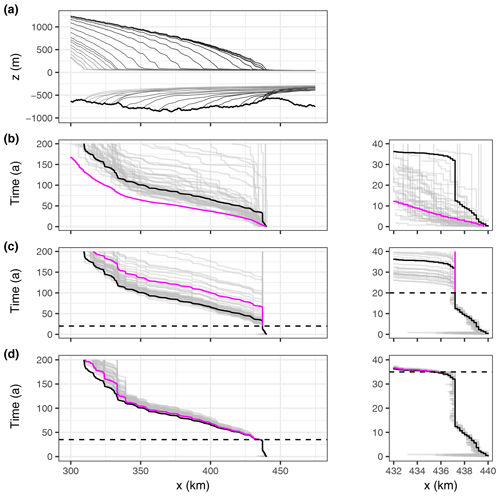Figure 2(a) Reference ice sheet topography every 10 years from t=0 to t=200  a (black to grey). (b)(d) GL position as a function of the simulation time for the reference (black line), for the ensemble (grey lines), and for the deterministic forecast (magenta line) (b) without assimilation, (c) with assimilation up to t=20  a and (d) with assimilation up to t=35  a. The right column shows a zoom on the first 40 years. In (c)(d), the horizontal dashed line shows the end of the assimilation window.

For the basal friction, we use a synthetic sinusoidal function with two wavelengths for C ($\mathrm{MPa}\phantom{\rule{0.125em}{0ex}}{\mathrm{m}}^{-\mathrm{1}/\mathrm{3}}\phantom{\rule{0.125em}{0ex}}{\mathrm{a}}^{\mathrm{1}/\mathrm{3}}$)

$\begin{array}{}\text{(21)}& C=\mathrm{0.020}+\mathrm{0.015}\phantom{\rule{0.25em}{0ex}}\text{sin}\phantom{\rule{0.25em}{0ex}}\left(\mathrm{5}\frac{\mathrm{2}\mathit{\pi }x}{L}\right)\phantom{\rule{0.25em}{0ex}}\text{sin}\phantom{\rule{0.25em}{0ex}}\left(\mathrm{100}\frac{\mathrm{2}\mathit{\pi }x}{L}\right),\end{array}$

with L=800 km (Fig. 3).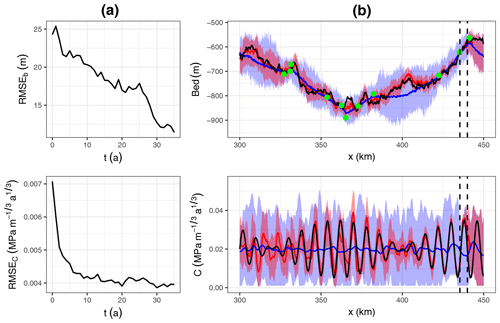Figure 3(a) RMSE between the reference and the analysed ensemble mean for the bed and friction coefficient. (b) For the bed and friction coefficient, the reference is shown in black, the synthetic bed measurements in the top panel are shown as green dots, the ensemble mean before assimilation is in blue and at t=35 a in red. The shading shows the ensemble spread between the minimum and maximum values, before assimilation (blue) and at t=35 a (red). The dashed vertical lines show the GL position at t=0 and t=35 a.

While not tuned to match any specific glacier, this synthetic design compares relatively well to the conditions found in Thwaites Glacier (Antarctica). Thwaites has been the focus of many recent studies as it is undergoing rapid ice loss and, connected to deep marine-based basins, its retreat could trigger a large-scale collapse of the West Antarctic Ice Sheet over the next centuries . In Fig. 4, C and b are compared with model results from along three streamlines. In , C has been inferred from the observed surface velocities using a time-independent control inverse method and a SSA model. We can see that our synthetic design is realistic in terms of both amplitude and spatial variations. As the other characteristics (geometry, small flow divergence and convergence) are also similar, the model velocities have a good order of magnitude.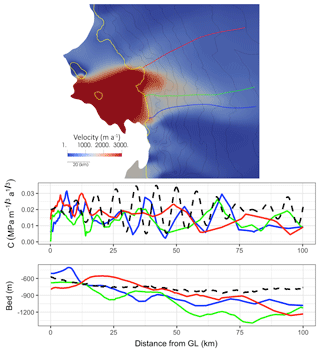Figure 4Thwaites Glacier (Antarctica). Model results from : model velocities (top) and friction coefficient C and bed elevation b extracted along three streamlines (same colour code). Synthetic values used in this study are shown with black dashed lines. Note that the mesh resolution varies from 200 m close to the GL, shown in yellow in the top panel, to 10 km at the upstream end of the streamlines.

Using a uniform ice rigidity $B=\left(\mathrm{2}A{\right)}^{-\mathrm{1}/n}=\mathrm{0.4}\phantom{\rule{0.125em}{0ex}}\mathrm{MPa}\phantom{\rule{0.125em}{0ex}}{\mathrm{a}}^{\mathrm{1}/\mathrm{3}}$, we grow an ice sheet to steady-state using a uniform surface accumulation ${a}_{\mathrm{s}}=\mathrm{0.5}\phantom{\rule{0.125em}{0ex}}\mathrm{m}\phantom{\rule{0.125em}{0ex}}{\mathrm{a}}^{-\mathrm{1}}$ and no basal melting ab=0. The steady state GL is located at x=440 km, just downstream of the region of overdeepening (Fig. 2).

In , observed ice flow accelerations in the Amundsen Sea sector have been attributed to the decadal oceanic variability, where warm phases associated with increased basal melt induce a thinning of the ice shelves, reducing their buttressing effect and initiating short-lived periods of unstable retreat of the most vulnerable GLs. In a flow line experiment the ice shelf does not exert any buttressing effect. Using a suite of melting and calving perturbation experiments for Pine Island Glacier, have shown that, when initiated, the dynamics of the unstable retreat are fairly independent of the type and magnitude of the perturbation. Here, to trigger the initial acceleration, we instantaneously decrease the ice rigidity to $B=\mathrm{0.3}\phantom{\rule{0.125em}{0ex}}\mathrm{MPa}\phantom{\rule{0.125em}{0ex}}{\mathrm{a}}^{\mathrm{1}/\mathrm{3}}$ at t=0, keeping all the other parameters constant.

This initial perturbation induces an acceleration, a thinning and a retreat of the GL. The model is then run for 200 years with a time step d$t=\mathrm{5}\phantom{\rule{0.125em}{0ex}}{\mathrm{10}}^{-\mathrm{3}}\phantom{\rule{0.125em}{0ex}}{\mathrm{a}}^{-\mathrm{1}}$. After a short stabilisation at x=437.2 km between t=13 a and t=32 a, the GL retreats at a rate of approximately 1 km a−1 during the following 100 years, and then the rate decreases as the GL enters an area of down-slopping bed (Fig. 2). The retreat rate shows small variations associated with spatial variations in the topography and basal friction.

## 3.2 Synthetic observations

From the reference run, we generate synthetic noisy observations that are typical of the resolution and performance of actual observing systems.

For the bed, we mimic an airborne radar survey conducted perpendicular to the ice flow with an along-flow resolution of approximately 15 km. For this, we randomly select 54 locations between x=0 and x=800 km and then linearly interpolate the true bed and add a random uncorrelated Gaussian noise with a standard deviation ${\mathit{\sigma }}_{\mathrm{b}}^{\mathrm{obs}}=\mathrm{20}\phantom{\rule{0.125em}{0ex}}\phantom{\rule{0.125em}{0ex}}\mathrm{m}$ (Fig. 3).

We assume that the surface elevation and velocities are observed at an annual resolution at each mesh node. We then add an uncorrelated Gaussian noise with a standard deviation ${\mathit{\sigma }}_{{z}_{\mathrm{s}}^{\mathrm{obs}}}=\mathrm{10}\phantom{\rule{0.125em}{0ex}}\mathrm{m}$ for the surface elevation and ${\mathit{\sigma }}_{\mathrm{u}}^{\mathrm{obs}}=\mathrm{20}\phantom{\rule{0.125em}{0ex}}\phantom{\rule{0.125em}{0ex}}\mathrm{m}\phantom{\rule{0.125em}{0ex}}{\mathrm{a}}^{-\mathrm{1}}$ for the velocity. The most recent velocity products are now posted with a monthly to annual resolution . The reported uncertainty for individual velocity estimates using the 6 and 12 d image pairs from the Sentinel-1A/B satellites is 6.2 and 17.5 m a−1 for the two horizontal velocity components in stable conditions; however this could be underestimated in the coastal areas. For the surface elevation, the spatial and temporal resolution as well as the coverage and uncertainty will depend on the sensors. The ArcticDEM (http://arcticdem.org, last access: 31 January 2019) is a collection of openly available digital surface models derived from satellite imagery and posted at 2 m spatial resolution. After co-registration, a standard deviation ranging from 2 to 4 m has been reported for the uncertainty of elevation difference between two individual models of static surfaces . Using the same satellites, Greenland digital elevation models are now posted with a 3-month temporal resolution (https://nsidc.org/data/nsidc-0715, last access: 28 August 2019).

## 3.3 Assimilation setup

We recall that our aim is to initialise the model using the DA framework to estimate the state together with the basal conditions. As a simplification to realistic experiments, we assume in the following that the ice rheological properties (represented by the Glen flow law and its parameters) and the forcing (represented by the surface and basal mass balances in Eq. 4) are perfectly known. In addition, we assume that the form of the basal friction follows Eq. (3) with $m=\mathrm{1}/\mathrm{3}$, so that only the spatially varying friction coefficient C is uncertain.

In our model, as the force balance Eq. (1) contains no time derivative, the velocity is a diagnostic variable. Because of the flotation condition, the topography can be represented by only one prognostic variable. The state vector x is then given by the free surface elevation zs at every mesh node, and we use the floatation Eq. (5) for the mapping between the ice thickness H and zs. The state vector is augmented by the two parameters to be estimated, the bedrock topography b and the basal friction coefficient C. For the parameters we assume a persistence model, i.e. no time evolution, during the forecast step (Eq. 8). Because the velocities are insensitive to the basal conditions where ice is floating, these two parameters are included in the state vector only for the nodes where at least one member is grounded. In addition, to insure that C remains positive, we use the following change of variable for the assimilation C=α2. Although it does not insure uniqueness of the estimation as α and α would lead to the same C, this change of variable is classical (MacAyeal1993) and was chosen as the reference friction coefficient spans only 1 order of magnitude. Similar performances were found using the other classical change of variable C=10α as in .

Because both zs and b are included in the state vector, the analysis does not conserve the ice sheet volume, for either the ensemble mean or the individual members. However, as illustrated in Fig. 1, the estimation of zs and b, and thus of the ice thickness, should be improved at each analysis as more data are assimilated, and the final state is the best estimation provided by the filter knowing the model, all the observations during the assimilation window and their uncertainties. As mentioned in the introduction, if the main interest is an analysis of past volume changes, a smoother or a variational method might be more appropriate. The smoother extension of the ESTKF can be found in . Note however that, interestingly, if we expect that the filter will improve the estimation of the ice thickness, there is no guaranty in general that it will provide a better estimate of the total volume as an a priori state with a totally different thickness distribution could lead, by compensation of the errors, to a perfect estimate of the true volume.

Kalman-based filters are based on the hypothesis of the independence between the background, i.e. the initial ensemble, and the observations that are used during the assimilation. As the synthetic bed observations will be used to construct the initial ensemble (see next section), we assimilate only the surface elevation and velocity observations, every year from t=1 a up to t=35 a. The observation operator is a simple mapping for the surface elevation and is given by the non-linear SSA equation (Eq. 1) for the surface velocities.

Finally, to illustrate the effect of the transient assimilation on model projections on timescales relevant for sea level projections, the analysed states at t=20 a and t=35 a are used to run deterministic and ensemble forecasts up to t=200 a. The deterministic forecast uses the ensemble mean produced by the analysis while the ensemble forecast propagates the full ensemble.

## 3.4 Initial ensemble

For atmosphere and ocean models, the initial state is usually sampled from a climatology, either observed or from a model run. This method can not be used for the parameters and the initial ensemble must reflect the background and the estimation of its uncertainty, available a priori before the assimilation. Following previous studies , we assume that the initial distributions for b and C are Gaussian with a given mean and a prescribed covariance model. Furthermore we assume no cross-correlation between the initial b, C and zs, and we draw the initial ensembles independently.

For b and C, the initial samples are drawn using the R package gstat . As is classical in geostatistics, the covariance model is prescribed using a variogram γ(d) that is half the variance of the difference between field values as a function of their separation d. It is usually defined by two parameters, the sill s that defines the semi-variance at large distances and the range ra which, for asymptotic functions, is defined as the distance where the γ(ra)=0.95s. The package gstat allows one to directly draw simulations, i.e. random realisations of the field, from the prescribed spatial moments .

For the bed we use an exponential function,

$\begin{array}{}\text{(22)}& \mathit{\gamma }\left(d\right)=s\left(\mathrm{1}-{e}^{-\frac{\mathrm{3}d}{{r}_{\mathrm{a}}}}\right),\end{array}$

with ra=50 km and s=4000 m2. We also add a nugget model defined by

with nug=200 m2. This model is meant to represent the bed measurement error. To draw the initial ensemble, the simulations are conditioned with the bed observations. This procedure gives an initial ensemble that is drawn from the posterior probability distribution that would be obtained using ordinary kriging with the same observations and variograms. The ensemble mean and spread for a 50-member ensemble are shown in Fig. 3 and the first three members are shown in Fig. 5. As expected, the ensemble spread increases with the distance from the observations. At the observation locations, the spread is controlled by the nugget. For the individual members, the nugget controls the small-scale variability, resulting in a roughness larger than the reference. When averaged this roughness disappears, and the ensemble mean has a much smoother topography.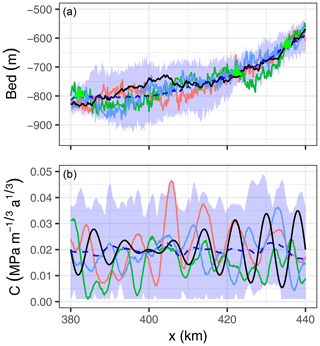Figure 5For the initial ensemble for the bed and friction coefficient, the ensemble mean is the dashed blue curve, and the shading shows the ensemble spread. Coloured solid lines show the first three members. The reference is shown in black and the synthetic bed measurements are shown as green triangles.

For the friction coefficient, we assume that we know the mean value ${C}_{\mathrm{mean}}=\mathrm{0.020}\phantom{\rule{0.125em}{0ex}}\mathrm{MPa}\phantom{\rule{0.125em}{0ex}}{\mathrm{m}}^{-\mathrm{1}/\mathrm{3}}\phantom{\rule{0.125em}{0ex}}{\mathrm{a}}^{\mathrm{1}/\mathrm{3}}$ and draw unconditional simulations. For the spatial dependence, we use a Gaussian function $\mathit{\gamma }\left(d\right)=s\left(\mathrm{1}-{e}^{-\mathrm{3}\left(\frac{d}{{r}_{\mathrm{a}}}{\right)}^{\mathrm{2}}}\right)$ for the variogram using a range ra=2.5 km and a sill $s={\mathrm{8.10}}^{-\mathrm{5}}\phantom{\rule{0.125em}{0ex}}{\mathrm{MPa}}^{\mathrm{2}}\phantom{\rule{0.125em}{0ex}}{\mathrm{m}}^{-\mathrm{2}/\mathrm{3}}\phantom{\rule{0.125em}{0ex}}{\mathrm{a}}^{\mathrm{2}/\mathrm{3}}$. This results in initial ensemble members that have approximately the same maximal amplitude as the reference, as shown in Fig. 5.

For the free surface, we initialise all the members using the observed (noisy) free surface at t=0. Doing so, we implicitly assume that the spread of the ensemble induced by the uncertain initial conditions at the first analysis is small compared to the spread induced by the uncertain parameters. This is motivated by the fact that divergence anomalies induced by uncertainties in model parameters can typically reach tens to hundreds of metres per year in fast-flowing areas .

4 Results

## 4.1 Assimilation

To assess the performance of the DA in retrieving the basal conditions, we compute the root-mean-square error (RMSE) between the analysed ensemble mean and the reference for both the bed and the friction coefficient, RMSEb and RMSEC. After each analysis, the RMSE is computed using all the nodes where the basal conditions have been updated by the assimilation, i.e. at least one member is grounded, and where x 300 km. The last value mentioned is close to the position reached by the grounding line after 200 years in the reference simulation; moreover, during the assimilation window, the reference velocity at this location is close to 80 m a−1 (Fig. 6), so that the relative noise is ∼25 % and we do not expect too much improvement from the DA upstream as the velocity tends to 0.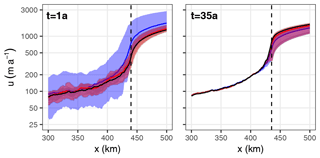Figure 6Velocity, u, at t=1 a and t=35 a. The reference is in black; the ensemble mean before and after the analysis is in blue and red, respectively. The shading shows the ensemble spread between the minimum and maximum. The dashed vertical black line indicates grounding line position.

Here the size of the state vector x, Nx, is approximately 8400, i.e. zs at every node and the basal conditions, b and C, in the grounded part. To test the performances of DA in conditions that would be numerically affordable for real applications, we run the assimilation with relatively small ensemble sizes Ne=30, Ne=50 and Ne=100. In this case, inflation and localisation are required to counteract the effects of undersampling and we test a range of forgetting factors ρ and localisation radius r. The errors obtained at t=20 a relative to the errors from the initial ensemble mean are shown in Fig. 7. The performances of the assimilation for Ne=50 and Ne=100 are very similar. The filter diverges and produces errors larger than the initial errors for a localisation radius r≤4 km. However, for larger localisation radii, the assimilation is relatively robust for a wide range of r and ρ, with errors reduced by ∼30 % for b and ∼40 % for C. Decreasing the ensemble sizes reduces the filter performance but there is still a reduction of the errors by ∼20 % and ∼30 %, respectively, with Ne=30. For the two smallest ensembles, there is an optimal value for r, and increasing r above this value decreases the filter performance. In general, this optimal value for r increases as ρ decreases, because the ensemble spread reduction induced by assimilating more observations is counterbalanced by the inflation.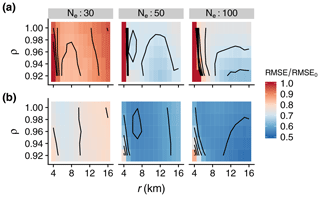Figure 7RMSE at t=20 a, relative to the initial (before assimilation) RMSE as a function of the forgetting factor ρ and the localisation radius r for different ensemble sizes Ne. (a) For the bed and (b) for the friction coefficient. Black lines show isovalues spaced by 5 %.

In the sequel we discuss the results obtained with an ensemble size Ne=50. As a compromise between the performances in retrieving b and C, we choose a forgetting factor ρ=0.92 and a localisation radius r=8 km. The evolution of the RMSEs as a function of assimilation time together with the initial and final ensembles are shown in Fig. 3. RMSEb decreases steadily from ∼25 m for the initial ensemble at t=0 to ∼12 m at t=35 a. For the basal friction, RMSEC is decreased by a factor of 1.75 during the first 10 years; then there is still a slight but much smaller improvement as new observations are assimilated.

At the end of the assimilation, for both fields, the spatial variations are well reproduced by the ensemble mean, and, compared to the initial ensemble, the difference from the reference is decreased everywhere except between 300 and 325 km for C. The reduction in the error is also accompanied by a diminution of the ensemble spread, represented by the minimum and maximum values in Fig. 3. This reduction is the most important just upstream of the grounding line where the relative noise for the velocity is the smallest. For the first 100 km upstream of the grounding line, the ensemble standard deviation increases by a factor of 4, from approximately 4 to 17 m for b and from $\mathrm{1}×{\mathrm{10}}^{-\mathrm{3}}$ to $\mathrm{4}×{\mathrm{10}}^{-\mathrm{3}}\phantom{\rule{0.125em}{0ex}}\mathrm{MPa}\phantom{\rule{0.125em}{0ex}}{\mathrm{m}}^{-\mathrm{1}/\mathrm{3}}\phantom{\rule{0.125em}{0ex}}{\mathrm{a}}^{\mathrm{1}/\mathrm{3}}$ for C. Downstream of the GL where all members are floating, the model is insensitive to the basal conditions and the initial ensemble is unchanged.

We expect that uncertainties in the ice sheet interior should not affect the short-term forecast of the coastal regions ; however for completeness we also show the results for the first 300 km in Fig. 8. For the bed there is only a small improvement of the ensemble mean with an RMSE decreasing from 50 to 45 m after 35 years. Because the relative observation error on the velocity is very high in the first kilometres, the reduction of the ensemble spread due to the assimilation of new observations is very small and eventually outperformed by the inflation, leading to an ensemble spread that becomes larger than before the assimilation. The model seems more sensitive to the basal friction and this effect is less pronounced for C with a continuous decrease in the RMSE and a small reduction of the ensemble spread everywhere.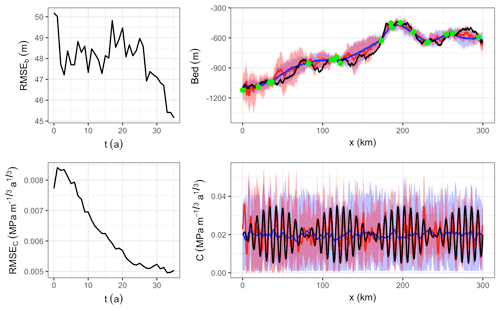Figure 8Same as Fig. 3 but with the RMSE computed for $x\in \left[\mathrm{0},\mathrm{300}\right]\phantom{\rule{0.125em}{0ex}}\mathrm{km}$.

Figure 2 shows that some members undergo a fast GL retreat of a few kilometres before assimilation at the end of the first year. Interestingly, as the assimilation updates both the thickness and the bed, it also corrects the GL position, which never departs by more than a few nodes from the reference for the rest of the assimilation period.

As in realistic simulations the true bed and friction are not available to assess the performance of the DA, we also look at the variables assimilated by the model. Figure 9 shows the RMSEs between the ensemble mean and the reference for the velocity u (RMSEu) and the free surface zs (${\text{RMSE}}_{{z}_{\mathrm{s}}}$), computed for the entire domain ($\mathrm{0}\le x\le \mathrm{800}\phantom{\rule{0.125em}{0ex}}\mathrm{km}$). We also report the evolution of the ensemble spread, computed as the square root of the averaged ensemble variance. The velocities before and after the analysis at t=1 and t=35 a are shown in Fig. 6. The RMSEs are largely decreased during the first few years, especially for the velocity with an error of more than 300 m a−1 before the first assimilation to approximately the noise level 20 m a−1, at t=20 a. For zs, ${\text{RMSE}}_{{z}_{\mathrm{s}}}$ is already below the noise level before the first analysis and decreases relatively steadily to reach ∼2 m after 35 years. RMSEu increases at the end of the period when the reference GL leaves the stable region. As can be shown in Fig. 6, the error is dominated by the larger difference over the ice shelf due to the few members that still have their GL at the stable location, largely affecting the ensemble mean.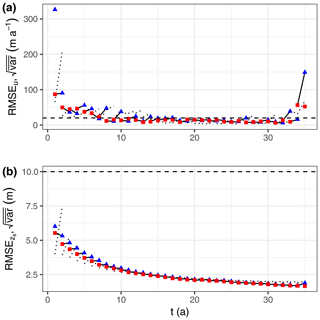Figure 9RMSE (solid lines) and square root of the averaged ensemble variance (dashed lines) during the assimilation window for (a) the velocity, u, and (b) the free surface, zs. Each year, the blue triangle and the red square are the RMSEs before and after the analysis, respectively. Each segment represents a 1-year forecast step.

In general, during the first and last years of the assimilation period, the error and the ensemble spread increase during the forecast step. The analysis step reduces both the error and the ensemble spread (Fig. 9). With the stabilisation of the grounding line, both the error and the spread remain relatively stable during the forecast, and as RMSEu and ${\text{RMSE}}_{{z}_{\mathrm{s}}}$ have already reached levels comparable to the observation noise, there is no much improvement during the analysis. After a few assimilation steps, as expected for a reliable ensemble, the error and the spread have similar values.

Similar conclusions are drawn if the assimilation is pursued up to t=50 a. Because of the sensitivity of the ice shelf velocities to the grounding line position, RMSEu shows a higher variability, but, with a few exceptions, stays close to the noise level. RMSEb and RMSEc stagnate as the reconstruction continually improves mostly in the first few tens of kilometres upstream of the GL where the relative noise on u is the smallest.

To assess the influence of the observation uncertainties in the performance of the DA, we repeat the experiment with the same localisation and inflation but different levels for the uncertainties on the observed surface velocity (${\mathit{\sigma }}_{\mathrm{u}}^{\mathrm{obs}}$) and surface elevation (${\mathit{\sigma }}_{{z}_{\mathrm{s}}}^{\mathrm{obs}}$) (see Sect. 3.2). We recall that these uncertainties are not correlated spatially and temporally. As shown in Fig. 10, the performance of the DA to retrieve both b and C increases when the uncertainty on the velocity observation ${\mathit{\sigma }}_{\mathrm{u}}^{\mathrm{obs}}$ decreases. However, when looking at the model velocities and surface elevation, this improvement is not significant as the RMSEs were already below the noise level. As shown in Fig. 11, as expected, decreasing ${\mathit{\sigma }}_{{z}_{\mathrm{s}}}^{\mathrm{obs}}$ improves the analysis for the surface elevation. However, it does not necessarily reflect on the basal conditions and, on the contrary, reducing ${\mathit{\sigma }}_{{z}_{\mathrm{s}}}^{\mathrm{obs}}$ below 10 m leads to an increase in RMSEC from 0.004 to $\mathrm{0.005}\phantom{\rule{0.125em}{0ex}}\mathrm{MPa}\phantom{\rule{0.125em}{0ex}}{\mathrm{m}}^{-\mathrm{1}/\mathrm{3}}\phantom{\rule{0.125em}{0ex}}{\mathrm{a}}^{\mathrm{1}/\mathrm{3}}$. However, again, this effect does not reflect on the model velocities that are retrieved with the same accuracy.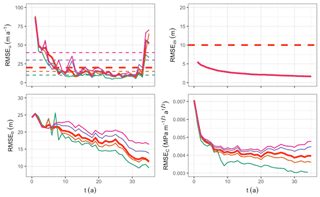Figure 10Sensitivity to the surface velocity observation error ${\mathit{\sigma }}_{\mathrm{u}}^{\mathrm{obs}}$ : RMSEs after each analysis, computed only for x≥300 km for b and C. The thick red lines correspond to the results with ${\mathit{\sigma }}_{\mathrm{u}}^{\mathrm{obs}}=\mathrm{20}\phantom{\rule{0.125em}{0ex}}\mathrm{m}{\mathrm{a}}^{-\mathrm{1}}$ and ${\mathit{\sigma }}_{{z}_{\mathrm{s}}^{\mathrm{obs}}}=\mathrm{10}\phantom{\rule{0.125em}{0ex}}\mathrm{m}$ shown in Figs. 3 and 9. The horizontal dashed lines correspond to the observation errors ${\mathit{\sigma }}_{\mathrm{u}}^{\mathrm{obs}}$ and the results are presented with solid lines using the same colour code. ${\mathit{\sigma }}_{{z}_{\mathrm{s}}^{\mathrm{obs}}}=\mathrm{10}\phantom{\rule{0.125em}{0ex}}\mathrm{m}$ for all the experiments.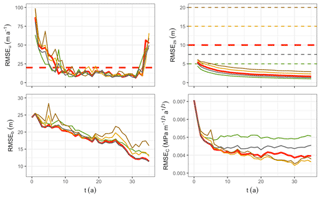Figure 11Sensitivity to the surface elevation observation error ${\mathit{\sigma }}_{{z}_{\mathrm{s}}^{\mathrm{obs}}}$: RMSEs after each analysis, computed only for x≥300 km for b and C. The thick red lines correspond to the results with ${\mathit{\sigma }}_{\mathrm{u}}^{\mathrm{obs}}=\mathrm{20}\phantom{\rule{0.125em}{0ex}}\mathrm{m}{\mathrm{a}}^{-\mathrm{1}}$ and ${\mathit{\sigma }}_{{z}_{\mathrm{s}}^{\mathrm{obs}}}=\mathrm{10}\phantom{\rule{0.125em}{0ex}}\mathrm{m}$ shown in Figs. 3 and 9. The horizontal dashed lines correspond to the observation errors ${\mathit{\sigma }}_{{z}_{\mathrm{s}}^{\mathrm{obs}}}$ and the results are presented with solid lines using the same colour code. ${\mathit{\sigma }}_{\mathrm{u}}^{\mathrm{obs}}=\mathrm{20}\phantom{\rule{0.125em}{0ex}}\mathrm{m}{\mathrm{a}}^{-\mathrm{1}}$ for all the experiments.

## 4.2 Forecast simulations

We now discuss model projections from the initial state to t=200 a.

Without assimilation, the deterministic forecast, i.e. using the ensemble mean basal conditions, rapidly leads to the fastest GL retreat, and after a few years the GL position is no longer included within the previsions from the ensemble (Fig. 2b). This is due to the fact that the ensemble mean is smoother than the reference and any of the ensemble members. The reference GL position is included in the ensemble, and at the end of the simulations most of the members are within ±25 km from the reference. However, for a few members the GL remains very stable near its initial position for tens to hundreds of years, eventually never switching to an unstable regime during the duration of the simulation. Retreat rates are relatively variable from one member to the other, depending on the basal conditions.

With assimilation, the ensemble mean is improved and the difference from the reference reduced. The deterministic forecast cannot be distinguished from the ensemble members any more (Fig. 2c–d). Retreat rates are closer to the reference, with the previsions from all the ensemble members being more or less parallel to the reference. We note however that when the forecast starts after an assimilation window of 20 years, i.e. during a period of stable GL position for the reference, the deterministic forecast leaves the stable position with a delay of approximately 25 years, and a few members remain stable for the entire simulation. On average between t=13 and t=32 a, the thinning rate at the GL in the reference simulation is approximately 0.6 m a−1, decreasing to 0.25 m a−1 during the last 2 years. The total thinning between two analyses is then much lower than the noise in the observed surface elevation and cannot be captured accurately by the DA. In addition, at the GL, the difference between the minimum and maximum bed elevation given by the ensemble is approximately 20 m. This remaining uncertainty induces a difference of more than 2 m for the floatation surface and combined with the small thinning rates explains the delays in the initiation of the instability.

Extending the assimilation window up to t=35 a when the reference has switched in a fast retreat allows the forcing of all the members in the unstable retreat. There is a very good agreement between the reference and the deterministic forecast up to t=110 a. This is also true for the ensemble, and after that the spread is larger and the predicted GLs are less retreated than for the reference.

These results can be summarised by looking at the distribution of the ensemble forecasts for the grounding line position and volume above floatation (VAF) at t=100 a in Fig. 12 where the relative VAF change is computed as $\left({\mathrm{VAF}}_{t=\mathrm{100}}-{\mathrm{VAF}}_{t=\mathrm{0}}^{\mathrm{ref}}\right)/{\mathrm{VAF}}_{t=\mathrm{0}}^{\mathrm{ref}}$, with ${\mathrm{VAF}}_{t=\mathrm{0}}^{\mathrm{ref}}$ the reference VAF at t=0. As expected there is a clear correlation between grounding line retreat and mass loss, with higher retreat leading to higher mass loss. The distributions are clearly non-Gaussian; however, even without assimilation there is already a mode close to the reference. The mode is more pronounced, with more members close to the reference as observations are assimilated. As discussed before, with no assimilation or a short assimilation up to t=20 a before the unstable retreat, the deterministic forecast can be very different from the mode of the ensemble forecast. However they are very similar if the assimilation is pursued up to t=35 a, within 1 % for the relative volume loss or 5 km for the GL position.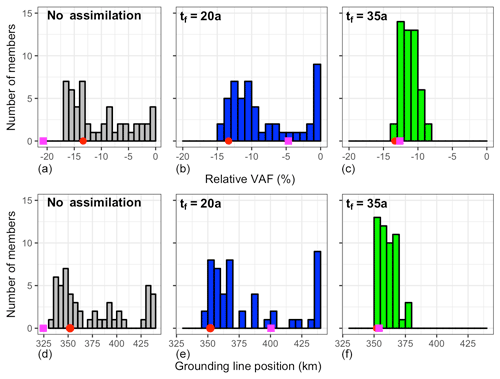Figure 12Ensemble forecast at t=100 a: (a)(c) relative change of volume above floatation (VAF) and (bottom) GL position with (a, b) no assimilation, (b, e) assimilation up to t=20 a and (c, f) assimilation up to t=35 a. The red circle correspond to the reference run and the magenta square to the deterministic forecast.

5 Discussion

Here, we have tested an ensemble Kalman filter to assimilate annually observed surface velocities and surface elevation in a marine ice sheet model. Similar to previous studies, we have shown that, in fast-flowing regions, it is possible to accurately separate and recover both the basal topography and basal friction from surface observations . In view of our results, because the synthetic bed observations were already used once to generate the initial ensemble, it seems unnecessary to assimilate these same observations again during each analysis as in .

Using a scheme that assimilates time-dependent observations provides a model state consistent with transient changes and that can directly serve as an optimal initial condition to run forecast simulations without the need of an additional relaxation . Interestingly the position of the grounding line is also corrected during the analysis step, and the ensemble quickly converges within a few grid nodes from the reference. In addition, the ensemble framework naturally allows the estimation and propagation of the uncertainty of the estimated parameters. Each assimilation of new data improves the reconstruction of the basal conditions (Fig. 3), and the first 10 years are very efficient in reducing error and the spread of the model surface velocities and elevation (Fig. 9). Furthermore, we have shown that the remaining uncertainties in the basal conditions do not significantly affect GL retreat rates once the unstable retreat is engaged. However, they can lead to considerable delays in the initiation of the instability. If the assimilation is pursued up to the beginning of the instability (35 years in our experiment) all the members exhibit the unstable retreat, and centennial-scale model projections converge to the reference (Fig. 12).

Good results have been obtained with relatively small ensembles (50 to 100 members) for a state vector of size Nx≈8400 and Ny=8002 observations. Similar to , we still see an improvement with a 30-member ensemble but the performances to retrieve the basal conditions are not as good. Running 2D plane view simulations with such ensemble sizes is largely possible as demonstrated by , who, using hybrid shallow ice–shallow shelf model, have run a 200-year ensemble forecast of the whole Antarctic Ice Sheet using 3000 members.

We have used inflation and localisation to stabilise the filter. The inflation giving the best results in (ρ=0.87–1.02) is similar to the values tested in this study. For the localisation radius r we have used values between 4 and 16 km, while they range from 80 to 120 km in . While this seems counter-intuitive as the velocities depend only on the local conditions with the shallow ice approximation used by , in fact, because we use a different grid size (dx=0.2 km compared to dx=5 km in ), for each node we assimilate twice as many observations. Our results are in agreement with the adaptive localisation radius proposed by . Using three different models, have shown that good performances are obtained when r is such that the effective local observation dimension, defined as the sum of the weights attributed to each observation during the local assimilation, is equal to the ensemble size. Here the observation weights decrease with the distance to the local assimilation domain following a fifth-order polynomial function mimicking a Gaussian function . The value r=8 km used for the 50-member ensemble gives an effective observation dimension of 56. Future studies should investigate if this result can be transposed to realistic 2D simulations with unstructured meshes.

In the experiments presented above, we have used a depth-integrated model for the force balance equations where GL migration is implemented through a hydrostatic floatation condition. This allows a full description of the ice topography with only one prognostic variable. Adaptation of the framework to a full-Stokes model requires minimum adaptations as these models do not rely on the floatation condition and solve a proper contact problem for the grounding line migration ; this implies incorporating the two prognostic free surfaces zb and zs in the state vector. These models might be more sensitive to unbalanced geometries that could result from the analyses, especially when localisation is used . However, the ESTKF, as the ETKF, induces a minimal transformation of the ensemble members and thus has better chances to preserve balance .

Before generalising such methods to real glacial systems, several points must be taken into consideration. They are independent of the DA method but they will eventually be treated differently in a variational or in an ensemble framework.

First, if the implementation is not an issue, the computational cost implied by running a full-Stokes model might remain a limiting factor. Compared to the Stokes solution, the SSA is known to overestimate the effects of bed topography perturbations on the surface profile for wavelengths less than a few ice thicknesses . How this issue can affect the reconstruction of the basal properties has never been quantified; however snapshot basal friction inversions have shown that the solution is sensitive to the force balance approximation . In addition, the MISMIP experiments have shown that the GL position and its response to a perturbation depend on the force balance solved by the models . In real applications, the performance of DA can be improved by explicitly taking into account the model error. Several strategies have been developed to account for this error, one approach with EnKFs being to use different versions of the model for different ensemble members . Further studies could investigate the potential benefits of using ensembles that combine several force balance approximations.

Second, the quality of the analysis and the accuracy of the error estimates depends on the observation error covariance matrix R. It is then important to provide meaningful error estimates. Recent velocity maps provide an error estimate reported as the 1σ value for each individual location . In general, this value agrees well with independent estimates; however care must be taken when the maps result from a composite of different sensors or different periods, and in general it might be difficult to properly estimate R.

A review paper by illustrates the impacts of badly calibrated observation and model error covariance matrices in a sequential DA framework and discusses available methods and challenges for their joint estimation. For the question of the impact of systematic errors, i.e. bias, either in the model or in the observations, and their correction by augmenting the system state in variational and ensemble DA, interested readers are referred to Dee (2005).

Third, the results depend on prior assumptions on the control variables and their variability, represented here by the initial ensemble. For the basal topography, current reference maps provide local error estimates ; however they do not provide information about spatial correlations so that generating initial ensembles with the correct statistics might be problematic. In addition, the gridding can result in a loss of information for some regions of dense measurements, or it can lead to too smooth terrains in sparsely sampled areas. With the aim of generating terrains that have the correct high-resolution roughness, propose a synthetic 100 m resolution Antarctic bed elevation that combines the reference topography of Bedmap2 with an unconditional simulation where the spatial correlation is fitted from dense radar measurements. This method could be used to generate initial ensembles but requires having access to the initial high-resolution measurements. Generating initial ensembles for the basal friction might be more problematic as there is in general no independent a priori information about the magnitude and spatial variability of the basal friction. If there is a correlation between the basal drag and the seismic observations of the bed conditions at a large scale, a proper physical theory is still missing to quantitatively incorporate such information in the models . It could be interesting to investigate how the existing multi-model basal friction reconstructions, based on snapshot inversions, could be used to derive initial uncertainty statistics and reduce the initial ensemble spread.

Finally, in our synthetic applications, we have not accounted for all potential sources of uncertainty which are, for example, as follows.

• The ice flow law. The ice viscosity depends on the englacial temperature, which itself is a function of the ice sheet history and the boundary forcing, including the geothermal heat flux . Several other processes also affect the ice viscosity, including damage and strain-induced mechanical anisotropy . For the stress exponent, if the value n=3 is used by most models, published values ranges between 1 and 5 (e.g. Gillet-Chaulet et al.2011).

• The friction law. More and more direct or indirect evidence shows that the friction under fast ice streams is at least partially controlled by the presence of sediments, leading to a Coulomb-type friction law . For hard beds, the development of subglacial cavities also implies deviations for the classical Weertman friction law .

• The density. The firn layer is not accounted for in most models; however its depth and density affect the floatation condition and thus the GL position (e.g. Griggs and Bamber2011). Directly assimilating the GL position, using, for example, the moving mesh approach developed by , would certainly be beneficial in realistic applications to reduce the discrepancy between the modelled and observed GL .

• The external forcings from the atmosphere and the ocean. Increasing mass loss rates from the ice sheets, in a large portion, can be attributed to a response to oceanic forcing, but multiple challenges remain for a proper assessment of their magnitude .

Realistic simulations with ice flow models cover a wide range of spatial and temporal scales, and the relative importance of these uncertainties as well as their representation in the models will certainly have to be evaluated partly on a case-by-case basis, requiring the development of a robust framework for a variety of applications.

6 Conclusions

Developing model initialisation strategies that properly reproduce the ice sheet dynamical mass losses observed over the last decades requires developing transient assimilation frameworks that are able to account for the growing availability of dense time series, especially from space observations. Here, we presented a synthetic twin experiment demonstrating the possibility of calibrating a marine ice model using an ensemble Kalman filter which requires fewer numerical developments than variational methods.

Using resolutions and noise levels consistent with current observing systems, good performances are obtained to recover both the basal friction and basal topography with an ensemble of at least 50 members. Localisation and inflation have been tuned manually; however the results are consistent over relatively wide ranges. Future studies should investigate how these values can be transposed to realistic applications. Nevertheless, there is an abundant and growing literature in other geophysical fields to overcome problems that we might be facing in future studies.

Once the GL enters an unstable region, retreat rates largely depend on the basal conditions; thus using DA to reduce the associated uncertainties largely increases the skill of the model to predict rates and magnitude of GL retreat for timescales relevant for sea level rise projections. In our simplified application, the assimilation of the surface observations was sufficient to capture the GL migration during the assimilation window, without explicitly assimilating the observed position. However, for the GL to enter an irreversible retreat, the thickness must reach a tipping point, i.e. the thickness at the GL must reach floatation. This can seriously impact the predictability of the system as, for small perturbations, remaining uncertainties on the basal conditions can lead to an uncertainty on the residence time of the GL on stabilisation points, which can be similar to the simulation timescale. However, if the assimilation is pursued up to a time when the glacier is engaged in unstable retreat, all the members exhibit instability, and the spread of centennial-scale model projections, in terms of volume and grounding line position, is largely reduced.

Finally, we have discussed the main challenges to tackle before generalising transient DA in ice sheet modelling. This includes a better assessment of the uncertainties in the model and in the observations used for the background and for the assimilation.

Code availability

Elmer/Ice code is publicly available through GitHub (https://github.com/ElmerCSC/elmerfem, last access: 25 June 2018, ). PDAF is distributed under the GNU General Public License, version 3, and is available at http://pdaf.awi.de (last access: 25 June 2018; ).

Appendix A: Notations

Table A1Notations and values used in this study associated with the ice flow model.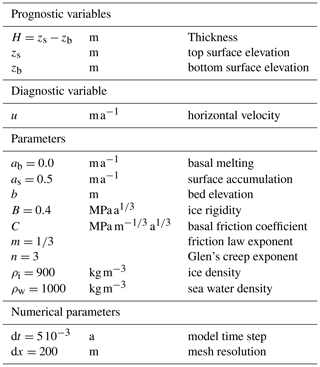Table A2Notations and values used in this study associated with the ensemble filter.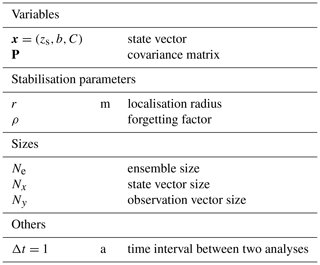Author contributions

FGC designed the experiments and wrote the paper.

Competing interests

The author declares that there is no conflict of interest.

Acknowledgements

The author thanks Gael Durand, Olivier Gagliardini and Jérémie Mouginot for valuable comments on the first drafts of the manuscript.

Financial support

This research has been supported by the French National Research Agency (ANR) through the TROIS-AS project (grant no. ANR-15-CE01-0005-01).

Review statement

This paper was edited by Carlos Martin and reviewed by Dan Goldberg and two anonymous referees.

References

Anderson, J. L. and Anderson, S. L.: A Monte Carlo implementation of the nonlinear filtering problem to produce ensemble assimilations and forecasts, Mon. Weather Rev., 127, 2741–2758, https://doi.org/10.1175/1520-0493(1999)127<2741:AMCIOT>2.0.CO;2, 1999. a

Bannister, R. N.: A review of operational methods of variational and ensemble-variational data assimilation: Ensemble-variational Data Assimilation, Q. J. Roy. Meteorol. Soc., 143, 607–633, https://doi.org/10.1002/qj.2982, 2017. a, b, c

Bishop, C. H., Etherton, B. J., and Majumdar, S. J.: Adaptive Sampling with the Ensemble Transform Kalman Filter. Part I: Theoretical Aspects, Mon. Weather Rev., 129, 420–436, https://doi.org/10.1175/1520-0493(2001)129<0420:ASWTET>2.0.CO;2, 2001. a, b

Bonan, B., Nodet, M., Ritz, C., and Peyaud, V.: An ETKF approach for initial state and parameter estimation in ice sheet modelling, Nonlin. Processes Geophys., 21, 569–582, https://doi.org/10.5194/npg-21-569-2014, 2014. a, b, c, d, e, f, g, h, i, j, k

Bonan, B., Nichols, N. K., Baines, M. J., and Partridge, D.: Data assimilation for moving mesh methods with an application to ice sheet modelling, Nonlin. Processes Geophys., 24, 515–534, https://doi.org/10.5194/npg-24-515-2017, 2017. a, b

Borstad, C. P., Rignot, E., Mouginot, J., and Schodlok, M. P.: Creep deformation and buttressing capacity of damaged ice shelves: theory and application to Larsen C ice shelf, The Cryosphere, 7, 1931–1947, https://doi.org/10.5194/tc-7-1931-2013, 2013. a

Brinkerhoff, D.J., Aschwanden, A., and Truffer, M.: Bayesian Inference of Subglacial Topography Using Mass Conservation. Front. Earth Sci. 4, 1–15, https://doi.org/10.3389/feart.2016.00008, 2016 a

Brondex, J., Gagliardini, O., Gillet-Chaulet, F., and Durand, G.: Sensitivity of grounding line dynamics to the choice of the friction law, J. Glaciol., 63, 854–866, https://doi.org/10.1017/jog.2017.51, 2017. a, b

Brondex, J., Gillet-Chaulet, F., and Gagliardini, O.: Sensitivity of centennial mass loss projections of the Amundsen basin to the friction law, The Cryosphere, 13, 177–195, https://doi.org/10.5194/tc-13-177-2019, 2019. a, b, c, d

Burgers, G., van Leeuwen, P. J., and Evensen, G.: Analysis scheme in the ensemble Kalman filter, Mon. Weather Rev., 126, 1719–1724, https://doi.org/10.1175/1520-0493(1998)126<1719:ASITEK>2.0.CO;2, 1998. a

Carrassi, A., Bocquet, M., Bertino, L., and Evensen, G.: Data assimilation in the geosciences: An overview of methods, issues, and perspectives, WIREs Clim Change, 9, e535, https://doi.org/10.1002/wcc.535, 2018. a, b, c

Church, J. A., Clark, P. U., Cazenave, A., Gregory, J. M., Jevrejeva, S., Levermann, A., Merrifield, M. A., Milne, G. A., Nerem, R. S., Nunn, P. D., Payne, A. J., Pfeffer, W. T., Stammer, D., and Unnikrishnan, A. S.: Sea level change, in: Climate Change 2013: The Physical Science Basis. Contribution of Working Group I to the Fifth Assessment Report of the Intergovernmental Panel on Climate Change, edited by: Stocker, T. F., Qin, D., Plattner, G.-K., Tignor, M., Allen, S. K., Boschung, J., Nauels, A., Xia, Y., Bex, V., and Midgley, P. M., Cambridge University Press, Cambridge, United Kingdom and New York, NY, USA, 2013. a

Cohn, S. E., da Silva, A., Guo, J., Sienkiewicz, M., and Lamich, D.: Assessing the Effects of Data Selection with the DAO Physical-Space Statistical Analysis System, Mon. Weather Rev., 126, 2913–2926, https://doi.org/10.1175/1520-0493(1998)126<2913:ATEODS>2.0.CO;2, 1998. a

Cosme, E., Verron, J., Brasseur, P., Blum, J., and Auroux, D.: Smoothing problems in a Bayesian framework and their linear Gaussian solutions, Mon. Weather Rev., 140, 683–695, 2012. a

Dai, C. and Howat, I. M.: Measuring Lava Flows With ArcticDEM: Application to the 2012–2013 Eruption of Tolbachik, Kamchatka, Geophys. Res. Lett., 44, 12133–12140, https://doi.org/10.1002/2017GL075920, 2017. a

Dee, D. P.: Bias and data assimilation, Q. J. Roy. Meteorol. Soc., 131, 3323–3343, https://doi.org/10.1256/qj.05.137, 2005. a

Durand, G. and Pattyn, F.: Reducing uncertainties in projections of Antarctic ice mass loss, The Cryosphere, 9, 2043–2055, https://doi.org/10.5194/tc-9-2043-2015, 2015. a

Durand, G., Gagliardini, O., Zwinger, T., Le Meur, E., and Hindmarsh, R. C.: Full Stokes modeling of marine ice sheets: influence of the grid size, Ann. Glaciol., 50, 109–114, https://doi.org/10.3189/172756409789624283, 2009. a, b

Durand, G., Gagliardini, O., Favier, L., Zwinger, T., and Meur, E. L.: Impact of bedrock description on modeling ice sheet dynamics, Geophys. Res. Lett., 38, L20501, https://doi.org/10.1029/2011GL048892, 2011. a, b, c

Enderlin, E. M., Howat, I. M., and Vieli, A.: High sensitivity of tidewater outlet glacier dynamics to shape, The Cryosphere, 7, 1007–1015, https://doi.org/10.5194/tc-7-1007-2013, 2013. a

Evensen, G.: Sequential data assimilation with a nonlinear quasi-geostrophic model using Monte Carlo methods to forecast error statistics, J. Geophys. Res., 99, 10143, https://doi.org/10.1029/94JC00572, 1994. a

Evensen, G. and van Leeuwen, P. J.: An ensemble Kalman smoother for nonlinear dynamics, Mon. Weather Rev., 128, 1852–1867, 2000. a

Favier, L., Durand, G., Cornford, S. L., Gudmundsson, G. H., Gagliardini, O., Gillet-Chaulet, F., Zwinger, T., Payne, A. J., and Le Brocq, A. M.: Retreat of Pine Island Glacier controlled by marine ice-sheet instability, Nat. Clim. Change, 4, 117–121, https://doi.org/10.1038/nclimate2094, 2014. a

Fournier, A., Fussell, D., and Carpenter, L.: Computer rendering of stochastic models, Commun. Assoc. Cornput. Mach., 25, 371–384, 1982. a

Fretwell, P., Pritchard, H. D., Vaughan, D. G., Bamber, J. L., Barrand, N. E., Bell, R., Bianchi, C., Bingham, R. G., Blankenship, D. D., Casassa, G., Catania, G., Callens, D., Conway, H., Cook, A. J., Corr, H. F. J., Damaske, D., Damm, V., Ferraccioli, F., Forsberg, R., Fujita, S., Gim, Y., Gogineni, P., Griggs, J. A., Hindmarsh, R. C. A., Holmlund, P., Holt, J. W., Jacobel, R. W., Jenkins, A., Jokat, W., Jordan, T., King, E. C., Kohler, J., Krabill, W., Riger-Kusk, M., Langley, K. A., Leitchenkov, G., Leuschen, C., Luyendyk, B. P., Matsuoka, K., Mouginot, J., Nitsche, F. O., Nogi, Y., Nost, O. A., Popov, S. V., Rignot, E., Rippin, D. M., Rivera, A., Roberts, J., Ross, N., Siegert, M. J., Smith, A. M., Steinhage, D., Studinger, M., Sun, B., Tinto, B. K., Welch, B. C., Wilson, D., Young, D. A., Xiangbin, C., and Zirizzotti, A.: Bedmap2: improved ice bed, surface and thickness datasets for Antarctica, The Cryosphere, 7, 375–393, https://doi.org/10.5194/tc-7-375-2013, 2013. a, b

Gagliardini, O., Cohen, D., Råback, P., and Zwinger, T.: Finite-element modeling of subglacial cavities and related friction law, J. Geophys. Res., 112, F02027, https://doi.org/10.1029/2006JF000576, 2007. a

Gagliardini, O., Zwinger, T., Gillet-Chaulet, F., Durand, G., Favier, L., de Fleurian, B., Greve, R., Malinen, M., Martín, C., Råback, P., Ruokolainen, J., Sacchettini, M., Schäfer, M., Seddik, H., and Thies, J.: Capabilities and performance of Elmer/Ice, a new-generation ice sheet model, Geosci. Model Dev., 6, 1299–1318, https://doi.org/10.5194/gmd-6-1299-2013, 2013. a, b

Gaspari, G. and Cohn, S. E.: Construction of correlation functions in two and three dimensions, Q. J. Roy. Meteorol. Soc., 125, 723–757, https://doi.org/10.1002/qj.49712555417, 1999. a, b

Gillet-Chaulet, F., Hindmarsh, R. C. A., Corr, H. F. J., King, E. C., and Jenkins, A.: In-situ quantification of ice rheology and direct measurement of the Raymond Effect at Summit, Greenland using a phase-sensitive radar, Geophys. Res. Lett., 38, L24503, https://doi.org/10.1029/2011GL049843, 2011. a

Gillet-Chaulet, F., Gagliardini, O., Seddik, H., Nodet, M., Durand, G., Ritz, C., Zwinger, T., Greve, R., and Vaughan, D. G.: Greenland ice sheet contribution to sea-level rise from a new-generation ice-sheet model, The Cryosphere, 6, 1561–1576, https://doi.org/10.5194/tc-6-1561-2012, 2012. a, b

Gillet-Chaulet, F., Durand, G., Gagliardini, O., Mosbeux, C., Mouginot, J., Rémy, F., and Ritz, C.: Assimilation of surface velocities acquired between 1996 and 2010 to constrain the form of the basal friction law under Pine Island Glacier, Geophys. Res. Lett., 43, 2016GL069937, https://doi.org/10.1002/2016GL069937, 2016. a

Gladstone, R. M., Payne, A. J., and Cornford, S. L.: Resolution requirements for grounding-line modelling: sensitivity to basal drag and ice-shelf buttressing, Ann. Glaciol., 53, 97–105, https://doi.org/10.3189/2012AoG60A148, 2012. a

Goldberg, D. N. and Heimbach, P.: Parameter and state estimation with a time-dependent adjoint marine ice sheet model, The Cryosphere, 7, 1659–1678, https://doi.org/10.5194/tc-7-1659-2013, 2013. a, b, c

Goldberg, D. N., Heimbach, P., Joughin, I., and Smith, B.: Committed retreat of Smith, Pope, and Kohler Glaciers over the next 30 years inferred by transient model calibration, The Cryosphere, 9, 2429–2446, https://doi.org/10.5194/tc-9-2429-2015, 2015. a, b, c

Goldberg, D. N., Narayanan, S. H. K., Hascoet, L., and Utke, J.: An optimized treatment for algorithmic differentiation of an important glaciological fixed-point problem, Geosci. Model Dev., 9, 1891–1904, https://doi.org/10.5194/gmd-9-1891-2016, 2016. a

Graham, F. S., Roberts, J. L., Galton-Fenzi, B. K., Young, D., Blankenship, D., and Siegert, M. J.: A high-resolution synthetic bed elevation grid of the Antarctic continent, Earth Syst. Sci. Data, 9, 267–279, https://doi.org/10.5194/essd-9-267-2017, 2017. a

Griggs, J. and Bamber, J.: Antarctic ice-shelf thickness from satellite radar altimetry, J. Glaciol., 57, 485–498, https://doi.org/10.3189/002214311796905659, 2011. a

Gudmundsson, G. H.: Analytical solutions for the surface response to small amplitude perturbations in boundary data in the shallow-ice-stream approximation, The Cryosphere, 2, 77–93, https://doi.org/10.5194/tc-2-77-2008, 2008. a

Gudmundsson, G. H. and Raymond, M.: On the limit to resolution and information on basal properties obtainable from surface data on ice streams, The Cryosphere, 2, 167–178, https://doi.org/10.5194/tc-2-167-2008, 2008. a, b

Hamill, T. M., Whitaker, J. S., and Snyder, C.: Distance-dependent filtering of background error covariance estimates in an ensemble Kalman filter, Mon. Weather Rev., 129, 2776–2790, https://doi.org/10.1175/1520-0493(2001)129<2776:DDFOBE>2.0.CO;2, 2001. a

Hascoët, L. and Morlighem, M.: Source-to-source adjoint Algorithmic Differentiation of an ice sheet model written in C, Opt. Method. Softw., 33, 829–843, https://doi.org/10.1080/10556788.2017.1396600, 2018. a

Hendricks Franssen, H. J., Kaiser, H. P., Kuhlmann, U., Bauser, G., Stauffer, F., Müller, R., and Kinzelbach, W.: Operational real‐time modeling with ensemble Kalman filter of variably saturated subsurface flow including stream‐aquifer interaction and parameter updating, Water Resour. Res., 47, W02532, https://doi.org/10.1029/2010WR009480, 2011. a

Houtekamer, P. L. and Mitchell, H. L.: Data assimilation using an ensemble Kalman filter technique, Mon. Weather Rev., 126, 796–811, https://doi.org/10.1175/1520-0493(1998)126<0796:DAUAEK>2.0.CO;2, 1998. a

Houtekamer, P. L. and Mitchell, H. L.: A Sequential Ensemble Kalman Filter for Atmospheric Data Assimilation, Mon. Weather Rev., 129, 123–137, https://doi.org/10.1175/1520-0493(2001)129<0123:ASEKFF>2.0.CO;2, 2001. a, b, c

Houtekamer, P. L., Mitchell, H. L., and Deng, X.: Model Error Representation in an Operational Ensemble Kalman Filter, Mon. Weather Rev., 137, 2126–2143, https://doi.org/10.1175/2008MWR2737.1, 2009. a

Houtekamer, P. L., Deng, X., Mitchell, H. L., Baek, S., and Gagnon, N.: Higher Resolution in an Operational Ensemble Kalman Filter, Mon. Weather Rev., 142, 1143–1162, https://doi.org/10.1175/MWR-D-13-00138.1, 2014. a

Hunt, B. R., Kostelich, E. J., and Szunyogh, I.: Efficient data assimilation for spatiotemporal chaos: A local ensemble transform Kalman filter, Physica D: Non. Phenom., 230, 112–126, https://doi.org/10.1016/j.physd.2006.11.008, 2007 a, b, c, d, e

Jenkins, A., Shoosmith, D., Dutrieux, P., Jacobs, S., Kim, T. W., Lee, S. H., Ha, H. K., and Stammerjohn, S.: West Antarctic Ice Sheet retreat in the Amundsen Sea driven by decadal oceanic variability, Nat. Geosci., 11, 733–738, https://doi.org/10.1038/s41561-018-0207-4, 2018. a

Joughin, I., Smith, B. E., and Holland, D. M.: Sensitivity of 21st century sea level to ocean-induced thinning of Pine Island Glacier, Antarctica, Geophys. Res. Lett., 37, L20502, https://doi.org/10.1029/2010GL044819, 2010. a, b

Joughin, I., Alley, R. B., and Holland, D. M.: Ice-Sheet Response to Oceanic Forcing, Science, 338, 1172–1176, https://doi.org/10.1126/science.1226481, 2012. a

Joughin, I., Smith, B. E., and Howat, I.: Greenland Ice Mapping Project: ice flow velocity variation at sub-monthly to decadal timescales, The Cryosphere, 12, 2211–2227, https://doi.org/10.5194/tc-12-2211-2018, 2018. a, b

Kalnay, E., Li, H., Miyoshi, T., Yang, S.-C., and Ballabrera-Poy, J.: 4-D-Var or ensemble Kalman filter?, Tellus A: Dynam. Meteorol. Oceanogr., 59, 758–773, https://doi.org/10.1111/j.1600-0870.2007.00261.x, 2007. a

Kirchgessner, P., Nerger, L., and Bunse-Gerstner, A.: On the Choice of an Optimal Localization Radius in Ensemble Kalman Filter Methods, Mon. Weather Rev., 142, 2165–2175, https://doi.org/10.1175/MWR-D-13-00246.1, 2014 a, b

Kyrke-Smith, T. M., Gudmundsson, G. H., and Farrell, P. E.: Can Seismic Observations of Bed Conditions on Ice Streams Help Constrain Parameters in Ice Flow Models?, J. Geophys. Res.-Earth Surf., 122, 2269–2282, https://doi.org/10.1002/2017JF004373, 2017 a

Larour, E., Morlighem, H. S. A. M., and Rignot, E.: Continental scale, high order, high spatial resolution, ice sheet modeling using the Ice Sheet System Model (ISSM), J. Geophys. Res., 117, F01022, https://doi.org/10.1029/2011JF002140, 2012. a

Larour, E., Utke, J., Csatho, B., Schenk, A., Seroussi, H., Morlighem, M., Rignot, E., Schlegel, N., and Khazendar, A.: Inferred basal friction and surface mass balance of the Northeast Greenland Ice Stream using data assimilation of ICESat (Ice Cloud and land Elevation Satellite) surface altimetry and ISSM (Ice Sheet System Model), The Cryosphere, 8, 2335–2351, https://doi.org/10.5194/tc-8-2335-2014, 2014. a

Larour, E., Utke, J., Bovin, A., Morlighem, M., and Perez, G.: An approach to computing discrete adjoints for MPI-parallelized models applied to Ice Sheet System Model 4.11, Geosci. Model Dev., 9, 3907–3918, https://doi.org/10.5194/gmd-9-3907-2016, 2016. a

Li, Z. and Navon, I. M.: Optimality of variational data assimilation and its relationship with the Kalman filter and smoother, Q. J. Roy. Meteorol. Soc., 127, 661–683, https://doi.org/10.1002/qj.49712757220, 2001 a, b

MacAyeal, D. R.: Large-scale ice flow over a viscous basal sediment: Theory and application to ice stream B, Antarctica, J. Geophys. Res.-Solid Earth, 94, 4071–4087, https://doi.org/10.1029/JB094iB04p04071, 1989. a

MacAyeal, D. R.: A tutorial on the use of control methods in ice-sheet modeling, J. Glaciol., 39, 91–98, 1993. a, b

Mitchell, H. L., Houtekamer, P. L., and Pellerin, G.: Ensemble size,balance, and model-error representation in an Ensemble Kalman Filter, Mon. Weather Rev., 130, 2791–2808, https://doi.org/10.1175/1520-0493(2002)130<2791:ESBAME>2.0.CO;2, 2002.. a

Morlighem, M., Rignot, E., Seroussi, H., Larour, E., Dhia, H. B., and Aubry, D.: Spatial patterns of basal drag inferred using control methods from a full-Stokes and simpler models for Pine Island Glacier, West Antarctica, Geophys. Res. Lett., 37, L14502, https://doi.org/10.1029/2010GL043853, 2010. a

Morlighem, M., Williams, C. N., Rignot, E., An, L., Arndt, J. E., Bamber, J. L., Catania, G., Chauché, N., Dowdeswell, J. A., Dorschel, B., Fenty, I., Hogan, K., Howat, I., Hubbard, A., Jakobsson, M., Jordan, T. M., Kjeldsen, K. K., Millan, R., Mayer, L., Mouginot, J., Noël, B. P. Y., O'Cofaigh, C., Palmer, S., Rysgaard, S., Seroussi, H., Siegert, M. J., Slabon, P., Straneo, F., van den Broeke, M. R., Weinrebe, W., Wood, M., and Zinglersen, K. B.: BedMachine v3: Complete Bed Topography and Ocean Bathymetry Mapping of Greenland From Multibeam Echo Sounding Combined With Mass Conservation, Geophys. Res. Lett., 44, 11051–11061, https://doi.org/10.1002/2017GL074954, 2017. a

Mosbeux, C., Gillet-Chaulet, F., and Gagliardini, O.: Comparison of adjoint and nudging methods to initialise ice sheet model basal conditions, Geosci. Model Dev., 9, 2549–2562, https://doi.org/10.5194/gmd-9-2549-2016, 2016. a

Mouginot, J., Scheuchl, B., and Rignot, E.: Mapping of Ice Motion in Antarctica Using Synthetic-Aperture Radar Data, Remote Sens., 4, 2753–2767, https://doi.org/10.3390/rs4092753, 2012. a, b

Mouginot, J., Rignot, E., Scheuchl, B., and Millan, R.: Comprehensive Annual Ice Sheet Velocity Mapping Using Landsat-8, Sentinel-1, and RADARSAT-2 Data, Remote Sens., 2017, 9, https://doi.org/10.3390/rs9040364, 2017. a

Murray, T.: Assessing the paradigm shift: deformable glacier beds, Quaternary Sci. Rev., 16, 996–1016, 1997. a

Nerger, L., Hiller, W., and Schröter, J.: A comparison of error subspace Kalman filters, Tellus A: Dynam. Meteorol. Oceanogr., 57, 715–735, https://doi.org/10.3402/tellusa.v57i5.14732a

Nerger, L., Hiller, W., and Schröter, J.: PDAF – The Parallel Data Assimilation Framework: Experiences with Kalman Filtering, in: Use of High Performance Computing in Meteorology, 63–83, WORLD SCIENTIFIC, Reading, UK, https://doi.org/10.1142/9789812701831_0006, 2005. a, b

Nerger, L., Danilov, S., Hiller, W., and Schröter, J.: Using sea-level data to constrain a finite-element primitive-equation ocean model with a local SEIK filter, Ocean Dynam., 56, 634–649, https://doi.org/10.1007/s10236-006-0083-0, 2006. a

Nerger, L., Schröter, J., and Hiller, W.: A Unification of Ensemble Square Root Kalman Filters, Mon. Weather Rev., 140, 2335–2345, https://doi.org/10.1175/MWR-D-11-00102.1, 2012. a, b, c, d, e

Nerger, L., Schulte, S., and Bunse-Gerstner, A.: On the influence of model nonlinearity and localization on ensemble Kalman smoothing, Q. J. Roy. Meteorol. Soc., 140, 2249–2259, https://doi.org/10.1002/qj.2293, 2014. a, b

Ott, E., Hunt, B. R., Szunyogh, I., Zimin, A. V., Kostelich, E. J., Corazza, M., Kalnay, E., Patil, D. J., and Yorke, A.: A local ensemble Kalman filter for atmospheric data assimilation, Tellus A, 56, 415–428, https://doi.org/10.1111/j.1600-0870.2004.00076.x, 2004. a

Pattyn, F., Huyghe, A., De Brabander, S., and De Smedt, B.: Role of transition zones in marine ice sheet dynamics, J. Geophys. Res., 111, F02004, https://doi.org/10.1029/2005JF000394,2006. a

Pattyn, F., Schoof, C., Perichon, L., Hindmarsh, R. C. A., Bueler, E., de Fleurian, B., Durand, G., Gagliardini, O., Gladstone, R., Goldberg, D., Gudmundsson, G. H., Huybrechts, P., Lee, V., Nick, F. M., Payne, A. J., Pollard, D., Rybak, O., Saito, F., and Vieli, A.: Results of the Marine Ice Sheet Model Intercomparison Project, MISMIP, The Cryosphere, 6, 573–588, https://doi.org/10.5194/tc-6-573-2012, 2012. a, b

Pattyn, F., Perichon, L., Durand, G., Favier, L., Gagliardini, O., Hindmarsh, R. C., Zwinger, T., Albrecht, T., Cornford, S., Docquier, D., FüRst, J. J., Goldberg, D., Gudmundsson, G. H., Humbert, A., Hütten, M., Huybrechts, P., Jouvet, G., Kleiner, T., Larour, E., Martin, D., Morlighem, M., Payne, A. J., Pollard, D., RüCkamp, M., Rybak, O., Seroussi, H., Thoma, M., and Wilkens, N.: Grounding-line migration in plan-view marine ice-sheet models: results of the ice2sea MISMIP3d intercomparison, J. Glaciol., 59, 410–422, https://doi.org/10.3189/2013JoG12J129, 2013. a, b

Pebesma, E. J. and Wesseling, C. G.: Gstat: a program for geostatistical modelling, prediction and simulation, Comput. Geosci., 24, 17–31, https://doi.org/10.1016/S0098-3004(97)00082-4, 1998. a, b

Pham, D. T., Verron, J., and Christine Roubaud, M.: A singular evolutive extended Kalman filter for data assimilation in oceanography, J. Mar. Sys., 16, 323–340, https://doi.org/10.1016/S0924-7963(97)00109-7, 1998. a, b, c, d

Pimienta, P., Duval, P., and Lipenkov, V. Y.: Mechanical behavior of anisotropic polar ice, Internationnal Association of Hydrological Sciences Publication 170 (Symposium at Vancouver 1987 – Physical Basis of Ice Sheet Modelling), 57–66, 1987 a

Pralong, M. R. and Gudmundsson, G. H.: Bayesian estimation of basal conditions on Rutford Ice Stream, West Antarctica, from surface data, J. Glaciol., 57, 315–324, https://doi.org/10.3189/002214311796406004, 2011 a

Ritz, C., Edwards, T. L., Durand, G., Payne, A. J., Peyaud, V., and Hindmarsh, R. C. A.: Potential sea-level rise from Antarctic ice-sheet instability constrained by observations, Nature, 528, 115–118, https://doi.org/10.1038/nature16147, 2015. a, b

Sakov, P. and Bertino, L.: Relation between two common localisation methods for the EnKF, Comput. Geosci. 15, 225–237, https://doi.org/10.1007/s10596-010-9202-6, 2011. a

Sakov, P., Counillon, F., Bertino, L., Lisaeter, K. A., Oke, P. R., and Korablev, A.: TOPAZ4: an ocean-sea ice data assimilation system for the North Atlantic and Arctic, Ocean Sci., 8, 633–656, https://doi.org/10.5194/os-8-633-2012, 2012. a

Scambos, T., Bell, R., Alley, R., Anandakrishnan, S., Bromwich, D., Brunt, K., Christianson, K., Creyts, T., Das, S., DeConto, R., Dutrieux, P., Fricker, H., Holland, D., MacGregor, J., Medley, B., Nicolas, J., Pollard, D., Siegfried, M., Smith, A., Steig, E., Trusel, L., Vaughan, D., and Yager, P.: How much, how fast?: A science review and outlook for research on the instability of Antarctica's Thwaites Glacier in the 21st century, Global Planet. Change, 153, 16–34, https://doi.org/10.1016/j.gloplacha.2017.04.008, 2017.  a, b

Schoof, C.: The effect of cavitation on glacier sliding, Proc. R. Soc. A, 461, 609–627, https://doi.org/10.1098/rspa.2004.1350, 2005. a

Schoof, C.: Marine ice-sheet dynamics. Part 1. The case of rapid sliding, J. Fluid Mech., 573, 27, https://doi.org/10.1017/S0022112006003570, 2007. a, b

Schulson, E. M. and Duval, P.: Creep and Fracture of Ice, Cambridge University Press, 2009. a

Seroussi, H., Morlighem, M., Rignot, E., Larour, E., Aubry, D., Dhia, H. B., and Kristensen, S. S.: Ice flux divergence anomalies on 79north Glacier, Greenland, Geophys. Res. Lett., 38, L09501, https://doi.org/10.1029/2011GL047338, 2011. a

Seroussi, H., Morlighem, M., Larour, E., Rignot, E., and Khazendar, A.: Hydrostatic grounding line parameterization in ice sheet models, The Cryosphere, 8, 2075–2087, https://doi.org/10.5194/tc-8-2075-2014, 2014. a, b

Sun, L., Seidou, O., Nistor, I., and Liu, K.: Review of the Kalman-type hydrological data assimilation, Hydrol. Sci. J., 61, 2348–2366, https://doi.org/10.1080/02626667.2015.1127376, 2016 a

Tandeo, P., Ailliot, P., Bocquet, M., Carrassi, A. Miyoshi, T., Pulido, M., and Zhen, Y.: A Review of Innovation-Based Methods to Jointly Estimate Model and Observation Error Covariance Matrices in Ensemble Data Assimilation, Mon. Weather Rev., submitted, 2020 a

Tsai, V. C., Stewart, A. L., and Thompson, A. F.: Marine ice-sheet profiles and stability under Coulomb basal conditions, J. Glaciol., 61, 205–215, https://doi.org/10.3189/2015JoG14J221, 2015. a

Tulaczyk, S., Kamb, W. B., and Engelhardt, H. F.: Basal mechanics of Ice Stream B, West Antarctica 1. Till mechanics, J. Geophys. Res., 105, 463–481, 2000. a

Van Liefferinge, B. and Pattyn, F.: Using ice-flow models to evaluate potential sites of million year-old ice in Antarctica, Clim. Past, 9, 2335–2345, https://doi.org/10.5194/cp-9-2335-2013, 2013. a

Vetra-Carvalho, S., van Leeuwen, P. J., Nerger, L., Barth, A., Altaf, M. U., Brasseur, P., Kirchgessner, P., and Beckers, J.-M.: State-of-the-art stochastic data assimilation methods for high-dimensional non-Gaussian problems, Tellus A: Dynam. Meteorol. Oceanogr., 70, 1445364, https://doi.org/10.1080/16000870.2018.1445364, 2018. a, b, c

Vieli, A. and Payne, A. J.: Application of control methods for modelling the flow of Pine Island Glacier, West Antarctica, Ann. Glaciol., 36, 197–204, 2003. a

Vieli, A. and Payne, A. J.: Assessing the ability of numerical ice sheet models to simulate grounding line migration, J. Geophys. Res.-Earth Surf., 110, F01003, https://doi.org/10.1029/2004JF000202, 2005. a